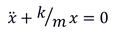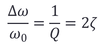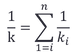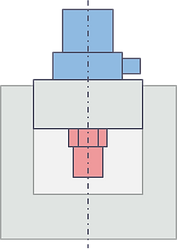a complex subject in a simple wayAccelerometer Properties

# Frequency-related Properties

## Basic Principles

When measuring vibrations in general, we can distinguish between two fundamentally different types, namely relative and absolute vibration measurement.

Relative vibration would be the measurement of a variable distance, for example the gap between a rotating shaft and a bearing. It can be measured by a proximity sensor installed on the bearing which is pointing at the shaft. This provides the motion of the shaft relative to the bearing.

An accelerometer (piezoelectric or other) is an inertial sensor and measures absolute vibration. The accelerometer measures vibration in space and not with respect to some other system. The inertial mass provides this characteristic because it is "suspended" by the sensing element in the sensor. The acceleration acts on the inertial mass and generates a force or movement of this mass which is measured by the sensing element. For absolute or seismic measurements either a velocity or acceleration sensor can be used but there are also absolute vibration sensors which measure displacement. In the case of a piezoelectric accelerometer we measure the force of the accelerated inertial mass. However the piezo sensing element is not completely rigid and therefore it allows for a tiny movement of the inertial mass. We will see in this chapter that this movement of the inertial mass is strongly dependent from the vibration frequency and leads to a most important frequency-related dynamic behavior.

## The Natural Frequency of the Seismic Transducer

We retain that

• Absolute vibration sensors show a frequency behaviour with a natural frequency

• Increasing the inertial mass m leads to a lower natural frequency while increasing the stiffness k of the suspension (spring) will increase it.

• The natural frequency of a piezoelectric accelerometer is

But beware: Strictly speaking this simple formula applies only to the natural frequency of an undamped oscillator however it is a good enough approximation for the natural frequency of a piezoelectric accelerometer.### Spring-Mass Oscillator without Damping

We look at our spring-mass oscillator model standing on a fixed base. The mass is m [kg] and the spring constant is k [N/m].

According to Sir Newton, the force acting on the mass due to the acceleration is . An additional force is due to the spring and is k·x. Since no other forces are involved we can write:orThe solution to this differential equation becomesThis is a continuous, harmonic oscillation with the frequency ω₀.is called the natural frequency of the system.Undamped spring-mass oscillator

### Spring-Mass Oscillator with Damping

The natural frequency of a damped oscillator is slightly different from that of the same oscillator without damping. The natural frequency of a damped oscillator is slightly different from that of the same oscillator without damping.A truly undamped oscillator does not exist in the real world but the damping of a piezoelectric accelerometer is very small and can practically be neglected. Other absolute vibration sensors (e.g. MEMS sensors or velocity sensors), show significant damping which must be taken into account.

For this reason and for the sake of completeness, both damped and undamped versions are mentioned here.

For more details please refer to the

For the calculation it is normally assumed that the frictional force is proportional to the velocity. In the illustration the damping is presented by the added "dashpot" with the damping coefficient c [N/m/s].

The damping force then becomes c ·   and the summation of all forces leads toHere it is useful to introduce the relative damping ζ which is  defined asUsing                       again, the differential equation can be written in the formSpring-mass oscillator with dampingDepending on the initial conditions, with x(t=0)=0 for example, the solution isThis is a sinusoidal oscillation with the frequency ωD and the initial amplitude A. The oscillation is decaying exponentially with the exponent - ζ· ω0 (see figure ).

The oscillation frequency ωis the damped natural frequency.

Withit is slightly different  from ω0 of the undamped oscillation depending on ζ .

For small ζ however (as we find in a piezoelectric accelerometer) we can safely calculate with ω0 instead of ωD.Decaying sinusoidal oscillation

## Frequency Characteristics of the Seismic Transducer

In this section we deal with inertial vibration sensors or seismic transducers in general. For piezoelectric accelerometers, we can use the mathematical model without damping, except for the region around the resonance. Most other inertial sensors, be it velocity or acceleration sensors, usually have significant damping that must be taken into account.

We retain that

•

•

### Inertial Sensor Frequency Response

As a model, we use the same damped oscillator. This time however, it does not stand still but moves with respect to the stationary space with the function u=g(t). Thus we force the oscillator to move and we speak of forced vibration.

A sensor that is built like this can measure vibration displacement, velocity or acceleration, depending on which part of the frequency range of the device is used. The division is made by the natural frequency. Below the natural frequency the vibration is measured in acceleration terms and in the upper frequency range as displacement, while around the natural frequency the sensor signal corresponds more to the velocity of the movement.

In the case of forced vibration we have the excitation u of the base and the relative motion x of the mass. The position of the mass m is given by u+x.

The acceleration force therefore becomesThe forces of spring and damping between base and mass are relative and remain the same as for the fixed oscillator. So we get for the equation of the equilibrium of the forces

orSpring-mass oscillator under forced vibration

On the right-hand side is the second derivative of the driving function, which means the acceleration of the base.

We therefore choose                               i.e. a harmonic oscillation with a constant acceleration amplitude UA.Using ω0 and ζ again as defined before, we obtain a similar differential equation as for the free oscillation, but it is this time inhomogeneous due to the term on the right:The solution of this equation consists of a first decaying part (as before in free oscillation) and a second stationary part, which follows the excitation u=g(t)

After a while the first part disappears. It remains the stationary part of the solution, the function of the displacement x of the mass with respect to the acceleration of the base:Here it is useful to introduce the dimensionless relative frequency ωR  i.e. the excitation frequency in relation to the natural frequencyIt is also common to use the frequency response representation (split into amplitude response and phase response), i.e. the ratio of the output amplitude to the input amplitude as a function of the excitation frequency.

The input represents an acceleration while the output in our model is a displacement.

The frequency response is therefore denoted by acceleration amplitude response ΦaThe dimension of the function is [s²] because it is [displacement X0 / acceleration UA].

The double logarithmic diagram shows the function for different values of the relative damping ζ .

The phase of the output is lagging behind the inputAcceleration amplitude responsePhase angle frequency response

### Resonance Frequency

​Resonance (Latin resonare "to echo") is the amplified oscillation of a vibratory system under an external, cyclic excitation close to the natural frequency of the system.

With each cycle the system absorbs more energy, which continuously increases the amplitude of the system until the energy absorption is in balance with the energy loss due to damping.

In sensor technology, resonance is simply defined as the maximum of the amplitude response to a constant input signal with varying frequency.

In fact we get, for considerable ζ-values, slightly different resonant frequencies for input signals with constant acceleration, velocity or displacement. ( With ζ = 0.1 the difference makes about 1% )

Acceleration resonance frequency:Velocity resonance frequency:Displacement resonance frequency:But how can it be that you get three different solutions for the same physical phenomenon?

#### Quality Factor Q and Bandwidth

The frequnecy response or transfer function of a single-mass oscillator can also be regarded as a bandpass filter. The amplification at resonance is then called quality factor Q and the width of the filter is called bandwidth B. Both Q and B depend on the damping. The damping of the oscillator can therefore be determined by recording the maximum response amplitude Фv.

The following relationship applies:The damping in a system is also characterised by the sharpness or width of the resonance peak.
The frequency difference Δω measured at -3dB from v)max is called the bandwidth ( -3dB corresponds to Q/2 ).
For the damping of the system we find for small values of ζQuality factor and bandwidth

## Accelerometer Natural Frequency / Resonance

Very often, especially in connection with piezoelectric accelerometers, the natural frequency is referred to as resonance, but strictly speaking resonance is something different. The two things are closely related but not identical.
The frequency at which a system would oscillate if there were no driving force and no damping force is called natural frequency.
While the natural frequency is an internal property of the system, resonance is the interaction with an external excitation at a frequency equal or close to its natural frequency. For systems with a considerable damping the resonance frequency is in fact slightly different from the natural frequency, whereas for a piezoelectric accelerometer the two values can be regarded as the same.

### Contributing design elements

We have seen that the natural frequency depends on the inertial mass and the spring constant. In a piezoelectric accelerometer, the "spring" is made up by the stack of piezoelectric elements.

The spring constant ki  of each part of the sensing element is given by the surface area Ai  divided by the thickness ti  times the Young’s modulus EiWith parts arranged in series and the total spring constant k becomesThus, for high stiffness, we prefer the smallest number of elements with a large surface area and small thickness. Besides the number of parts there are also the interfaces between them that reduce the stiffness. Therefore, attention must be paid to the flatness and surface quality of all parts.

### Mounted vs. Non-Mounted Natural Frequency or Resonance

When defining and calculating the natural frequency of the sensor (or oscillator), we assumed that the base is fixed in space. In the real world this is approximately the case when the sensor is mounted on a heavy and rigid body. In this case the vibration pattern has a node at the base and an antinode at the inertial mass. When the sensor is not mounted, the seismic mass and the base oscillate in counter action around a node located somewhere inside the sensor element. The natural frequency of the free, non-mounted sensor is therefore considerably higher than when it is mounted.
In order to say something about the high frequency capability of an accelerometer we need to look at the mounted condition. The non-mounted value can be used to check the conformity of a set of sensors, e.g. in a quality control.

### Measurement of the Natural Frequency / Resonance

The natural frequency of a piezoelectric accelerometer can be excited by a short mechanical impulse.

To measure f0 in the mounted state, we need a block with minimum ten times the weight of the sensor and a high Young's modulus. It is recommended to use a cube made of tungsten.

The following methods can be used to measure the mounted or non-mounted natural frequency.

A mechanical shock can be applied, for example, by a small metal ball attached to a thread. When striking with the suspended ball, make sure that it does not hit twice.

The Hsu-Nielsen test method is another possibility. This is a simple method in which a wide frequency range excitation signal is generated by breaking a mechanical pencil lead. Originally developed for testing acoustic emission sensors, the method is also excellent for testing the natural frequency of an accelerometerSteel ball impactHsu Nielsen source test method

After such an impact the inertial mass will be «ringing» at f0 and with a signal analizer or transient recording device we can read out f0. Basically, the damping rate can also be determined, although the signals need to be clean enough to do so.
We have to take into account that we are not only exciting the natural frequency of the sensing element, but also many resonance frequencies within the sensor. This means that we usually need a critical interpretation of the measurement result.

There is also a completely different method that uses the inverse piezoelectric effect to excite the natural / resonant frequency, by injecting an electrical signal. As injection signal we choose a single pulse or a alternatively a white noise. By calculating the FFT transfer function of the signal fed in via the sensor compared to the injection signal, the natural / resonant frequency can be easily determined.Signal injection test method

## Accelerometer Frequency Response

### Frequency response in the upper range

One of the principal features of a piezoelectric accelerometer is its frequency response. As we have seen above the frequency response function is depending on the resonance of the sensor.

The resonant frequency as such depends strongly on the construction of the sensor, but the evolution of the response curve is usually very close to the theoretically expected curve.

The figure shows the typical frequency response of a piezoelectric accelerometer. We find Q-factors of about 50 or even 100, which means ζ values in the order of 0.01 to 0.005.
For the frequency we are using the relation

As a rule of thumb, the response curve stays within 5% up to about 1/5 of the resonant frequency and the +3dB point is at about half the resonance frequency.

The phase shift is negligible below the resonance. At the resonance frequency however, it changes almost instantly to -180°.Typical accelerometer frequency response

### Frequency response in the lower range

Towards the lower frequencies, the correct reproduction of the acceleration signal is limited by the fact that the charge built up in the piezo element decays according to the time constant RC. The time constant itself is given by the capacitance C and the internal resistance R of the piezo element.

In the frequency domain, the RC element results in a high-pass filter with the cut-off frequencyBy using a charge amplifier, the time constant can be increased considerably and the cut-off frequency of the resulting high-pass filter can be reduced enormously. The time constant is then imposed by the charge converter, supposing the internal resistance of the piezo element is sufficient.

The detailed relations are shown in the chapter "Signal Conditioners".Typical single pole high-pass filter frequency response

The figure shows a typical characteristic for such a single pole high-pass filter. At the cut-off frequency fc we find an attenuation of -3 dB. If we search the -5% point we have to go to the region of about 10 times fc.

### Design and other Contributing Elements

Similar to the dynamic range of an accelerometer we find for the frequency response that the high end is rather defined trough the mechanical sensor design while the low end is rather given by the following electronics.

The frequency response at higher frequencies is determined by the resonance, therefore we have to consider the same points mentioned for the natural frequency. Furthermore, it must be taken into account that the mass of the accelerometer can influence the frequency behaviour of the measured object or create an additional resonance in the mechanical measuring path. This means the sensor with the lowest mass will normally be the best to measure high frequencies.

In addition to the sensor mass, the mounting surface of the accelerometer and the contact surface to the measured object are of particular importance.

### Measurement of the Frequency Response and Resonance

For measuring the frequency response, we basically use the same setup as for the calibration. I.e. a back-to-back mounting with a central block on which we mount the unit under test on one side and a reference transducer on the other side. Of course, the frequency response of the reference transducer also plays a role. Ideally, it should have a resonance that is about 10 times higher than the highest frequency to be measured. If this is not the case, the excitation amplitude must be adjusted accordingly. With a Laser reference this problem does not exist.

In addition, utmost attention must be paid to the surfaces of the central block. The flatness and roughness of these surfaces have a great influence on the resonance frequency. For very high frequencies, an oil film should be applied.

Unit under test (UUT)Reference

Accelerometer

Set-up for the frequency response measurement

To carry out a frequency response test we either set the drive signal to different fixed frequencies and read the signal of the UUT or we sweep the frequency of the drive signal slowly from the lowest to the highest frequency of interest.

By increasing the frequency until we reach the maximum output signal of the UUT, we measure the resonance. If we are interested in the Q-factor, care must be taken that the resonance peak is really hit by the excitation and remains long enough for the signal to rise to the actual maximum

#### Influence of the surface / coupling condition

The graph shows a series of real measurements of the resonance frequency of the same sensor mounted on a block with different surface finishes from ISO N3 (lapped) to N6 (ground). The resonance varies from 37.7 kHz down to 27.2 kHz for the dry mounted condition, which corresponds to a loss of 27 %!
With an oil film applied, the result is much better, but the influence of the surface is still visible.Measured values of the accelerometer resonance with different surface qualities and coupling conditions